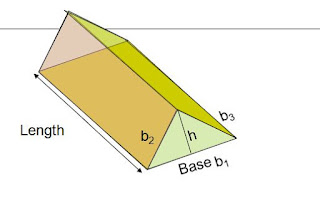# SCC Education

## RIGHT PRISM,Volume of Right Prism,Triangular Prism,formulas and solved example

A right prism is a solid which has two parallel planes of same
shape and size. Also, its lateral surface are perpendicular to its
parallel  sides.

Volume of Right Prism
Volume = Area of cross-section x Distance between parallel sides
= Base area x height
Triangular PrismVolume = Base area x height
= Triangle area x length of the solid
= ½ x base x height x length

Total surface area = Two triangles + three rectangles
= 2 x ½ x b x h  +  L x b1 + L x b2 + L x b3
= 2 x base area + (b1 + b2 + b3) x L
= 2 base area + Perimeter of the base x Length

Volume of a Prism

Volume = Base Area x Height
= ½ x 12 x 16 x 30
= 2880 cm3Perimeter of the base = 12 + 16 + 20
= 48 cm
T.S.A = 2 x Base Area + Perimeter of the base x height
= 2 x 96 + 48 x 30
= 1632 cm2.

Trapezoid

Volume = Base Area x Length
= ½ x (8 + 15) x 10 x 20
= 2300 cm3.

T.S.A = 2 x Base area + Perimeter of the base x height
= 1670 cm2.